Question
12 views

What is the parallax of a star that is 13.00  light-years away?

check_circle

Step 1

The parallax is an methond to calculate the distance between the objects in universe.

It is based on the trignometric concept.

Here, the parallax angle between (p) between the earth and nearby star is shown as,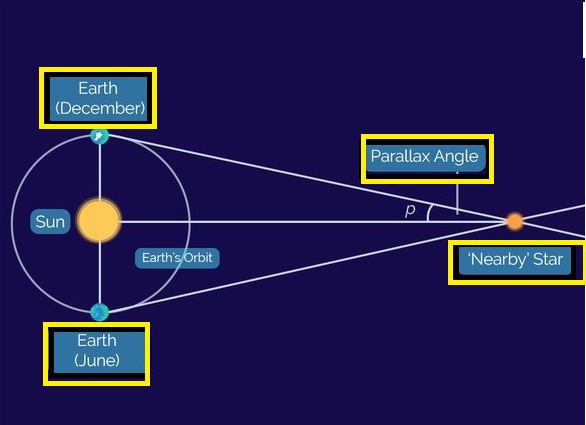The relation between the start distance and its parallax angle is;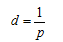Where,

d = distance between the earth and star.

p = parallax angle

3.2 Light yeras = 1 parsec

The parallax angle is used in the formula should be in arcseconds and distance should in parsec.

It is given in the question that the distance is 13 light years.

We have to find out the parallax of the star.

Step 2

First, we will convert the star distance unit from light years to parsec unit.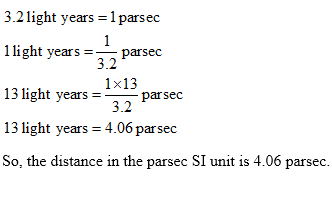Step 3

Now, we will find out the parallax angle.

The relation between the start distance and its parallax angle is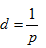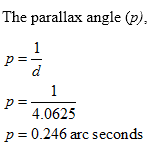...

### Want to see the full answer?

See Solution

#### Want to see this answer and more?

Solutions are written by subject experts who are available 24/7. Questions are typically answered within 1 hour.*

See Solution
*Response times may vary by subject and question.
Tagged in